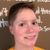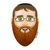## CodeNewbie Community 🌱 is a community of 11,555 amazing newbiesYechiel (he/him)

Posted on • Originally published at blog.yechiel.me

# Get Your (Fizz)Buzz On

## FizzBuzz In 3 Acts

Learning to code was full of learning moments. One that I look back upon fondly was when I did the Flatiron lesson on FizzBuzz. That memory has been greatly reinforced when Flatiron hired me as a Technical Coach; FizzBuzz was one of my favorite labs to help new students with.

I will explain why the process of learning (and then teaching) FizzBuzz stood out so much, but first a word on what FizzBuzz is.

## What The Fizz?

I know the first thing that comes to your mind when you hear FizzBuzz is probably something like this:FizzBuzz is a small game that interviewers use to weed out the extraordinarily bad coders from the ones who don’t know how to code at all. It’s a fairly simple problem, which anyone with the most rudimentary knowledge of how computers work should be able to solve, yet apparently, there are enough applicants for developer positions who can’t solve it to warrant its use in interviewing.

The problem is as follows:

Write a program that prints the numbers 1–100, but for every number that is a multiple of three print the word `Fizz`, for every number that’s a multiple of five print `Buzz`, and for every number that’s a multiple of both three and five print `FizzBuzz`. So the output for the first 15 numbers would look like this:
`1, 2, Fizz, 4, Buzz, Fizz, 7, 8, Fizz, Buzz, 11, Fizz, 13, 14, FizzBuzz…`

In this post, I will be showing the solutions in Ruby, just because I like Ruby, and I think it’s the prettiest language. But most of the concepts can be ported to any language.

## What Doesn’t Work

To explain the beauty of FizzBuzz, and why I love teaching it to new coders, let me demonstrate the first knee-jerk solution a new coder might come up with.

``````(1..100).each do |i|
if i % 3 == 0
puts "Fizz"
elsif i % 5 == 0
puts "Buzz"
elsif i % 3 == 0 && i % 5 == 0
puts "FizzBuzz"
else
puts i
end
end
``````

And in English:

For each number in the range of 1–100 check the following: if the number can be divided cleanly into three print `Fizz`, otherwise, if it can be divided cleanly into five print `Buzz`, otherwise, check if it can be divided cleanly into 3 AND 5 print `FizzBuzz`.

Seems pretty straightforward, no? If you gave those instructions to a human, you would be understandably annoyed if they messed it up. But apparently, that’s not clear enough for a computer. If you give the above code snippet to a computer, you would see a nice list of numbers, with plenty of Fizzes and Buzzes mixed in, but not one FizzBuzz. To understand why, we have to look at the difference between how humans look at instructions, and how computers do.

When a human looks at a block of code, we try to look at it as a whole entity. What is this code doing? What is it trying to accomplish? Why was it written? Computers don’t have that big-picture view; a computer will just analyze the code line by line and execute the instructions given.

Let’s try to look at the code above through the eyes of a computer.

First, the computer looks at the number 1. It takes it through the steps we outlined for it. Does it divide into 3? No. Okay, do nothing. Next, does it divide into 5? No. Okay, do nothing again. Next, does it divide into 3 and 5? No. Okay, do nothing. Next, print the number, got it.

The computer then repeats the process with number 2; it looks pretty much the same.

By number 3 the process is different. The computer tries dividing it into three; it works! Great! Let’s print Fizz. Next number, etc.

Now let’s take a look at what happens when we reach number 15. Remember, the computer only analyzes one line at a time, so the computer tries dividing 15 into 3, it works, the computer is happy, it prints Fizz and then goes right on to the next number, never reaching the line where its told to try dividing into 3 AND 5.

## The Solution

Fortunately, this can be fixed by simply moving the last condition to the front, so that the computer will try to divide the number into 3 and five before it tries them separately, like this:

``````(1..100).each do |i|

if i % 3 == 0 && i % 5 == 0
puts "FizzBuzz"
elsif i % 3 == 0
puts "Fizz"
elsif i % 5 == 0
puts "Buzz"
else
puts i
end
end
``````

## The More The Fuzzier

Now, as mentioned, FizzBuzz is a beginners problem, anyone who spent some time coding should be able to solve it without much thought. But in programming, there is never only one way to solve a problem.I would like to share a different way of solving FizzBuzz, one that looks a lot prettier in my opinion. This solution takes a slightly different approach:

``````(1..100).each do |i|

output = ""
output += "Fizz" if i % 3 == 0
output += "Buzz" if i % 5 == 0
output = i if output.empty?

puts output
end
``````

In English:

For each number in the range of 1–100 we start with an empty string (`""`), we then add `"Fizz"` to the string if the number is divisible by 3, we add `"Buzz"` to the string if the number is divisible by 5, if the string is still empty by this time (which will only happen if the number is not divisible by 3 or by 5) we will swap the empty string out for the number.

Notice how in this approach, we never check if the number is divisible by 3 AND 5, we just add Buzz to the existing string, which would be an empty string if the number is not divisible by three, and a string equaling Fizz if it is divisible by three.

## But Does It Scale?

So now we solved the problem, but as programmers, we always try to improve.

Our solution works, the problem is it doesn’t scale really well. Suppose we wanted to do the same thing but for all the numbers between 1 and 50? 500? 5000? We would have to write a separate FizzBuzz program for each of those.

The solution would, of course, be, to write a FizzBuzz method that we can provide with a number, and have it run the FizzBuzz logic on every number from one through the number we provide. We can even give it a default argument so that if we don’t provide a number it will print FizzBuzz for 1–100.

That would look like this:

``````def fizzbuzz(num = 100)
(1..num).each do |i|

output = ""
output += "Fizz" if i % 3 == 0
output += "Buzz" if i % 5 == 0
output = i if output.empty?

puts output
end
end
``````

There are many more ways to solve FizzBuzz in Ruby (and in every other language), feel free to add your favorite in the comments.

This article has been cross-posted from my blog Rabbi On Rails.
You can read more about my coding journey there, or by following me on Twitter @yechielk

## Top comments (2)Vicki Langer

This is really cool. I've never heard of this fizz buzz test, but it's kinda neat and I love the bunnies I love how you gave us different examples of how to solve this.Yechiel (he/him)

I've never actually gotten it in an interview, I think it's mostly a historical curiosity at this point, but we had it as a lab in bootcamp.